# 机器学习基石-数学篇：Why Can Machines Learn?

## Coursera | Machine Learning Foundations - Mathematical Foundations | Hsuan-Tien Lin

Thistledown    July 2, 2020

## 5 Training versus Testing

What we pay in choosing hypotheses during training: the growth function for representing effective number of choices …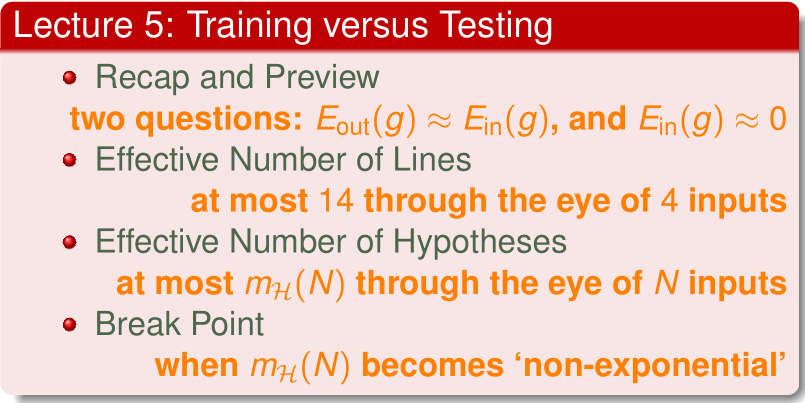#### 5.1 Recap and Preview

• Recap: the statistical learning flow

• if $\mathcal{H} = M$ finite, $N$ large enough
• for whatever $g$ picked by $\cal A$, $E_{out}(g) \approx E_{in}(g)$
• if $\cal A$ finds one $g$ with $E_{in}(g) \approx 0$
• PAC guarantee for $E_{out}(g) \approx 0$ $\Rightarrow$ learning possible
• Two central questions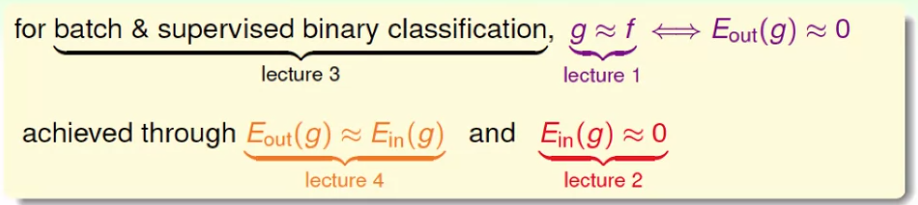• learning split to two central questions:
• can we make sure that $E_{out}(g)$ is close enough to $E_{in}(g)$?
• can we make $E_{in}(g)$ small enough?
• what role dose $M$ ($\vert \cal H \vert$) play for the two questions?
• Trade-off on $M$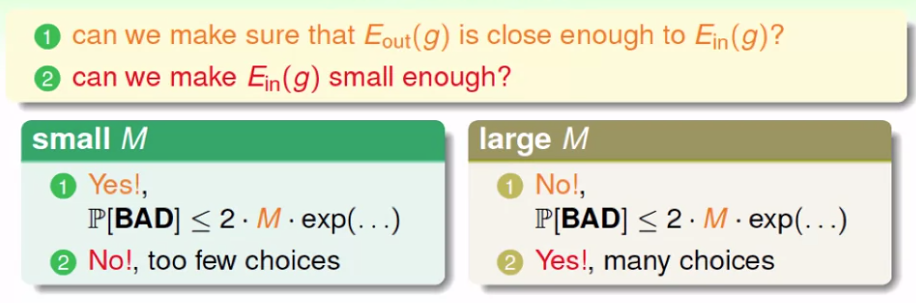• using the right $M$ (or $\cal H$) is important
• $M = \infty$ doomed?
• Preview

• Known

• Todo

• establish a finite quantity that replaces $M$

• justify the feasibility of learning for infinite $M$

• study $m_{\cal H}$ to understand its trade-off for right $\cal H$, just like $M$

• mysterious PLA to be fully resolved after 3 more lectures 🎉

QUIZ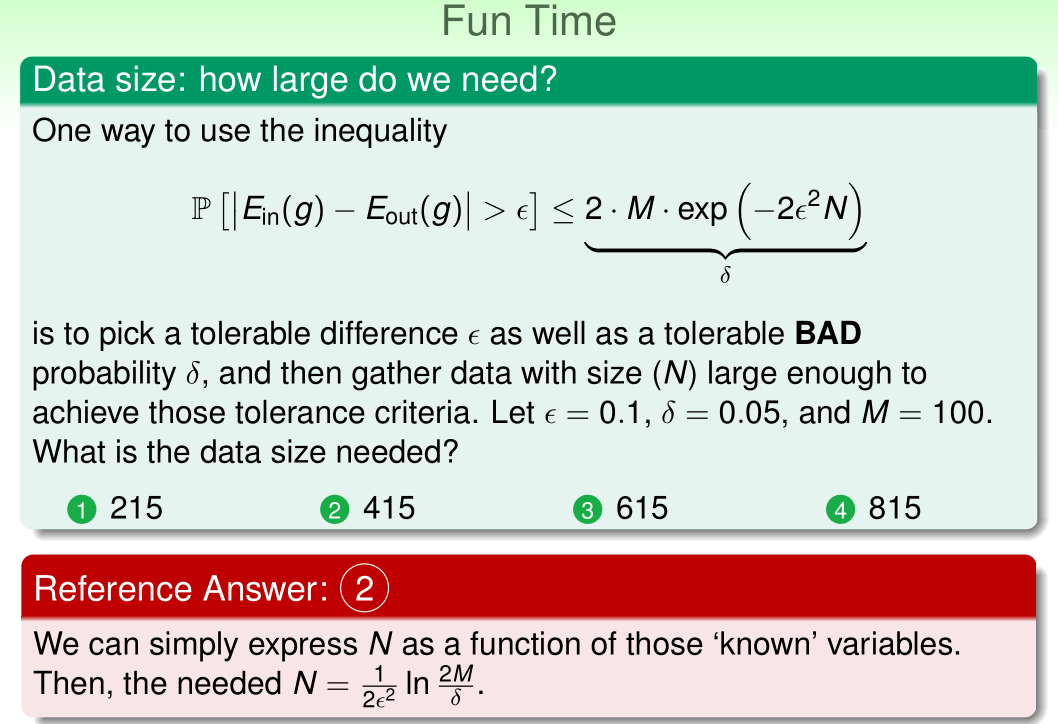#### 5.2 Effective Number of Lines

• Where did $M$ come from?

• BAD events $\mathcal{B}_m : \vert E_{in}(h_m) - E_{out}(h_m)\vert > \epsilon$

• to give $\cal A$ freedom of choice: bound $\Bbb{P}[\mathcal{B}_1 \text{ or } \cdots \text{ or } \mathcal{B}_M ]$

• worst case: all $\mathcal{B}_m$ non-overlapping

• where did uniform bound fail to consider for $M = \infty$?

• Where did uniform bound fail?

• BAD events $\mathcal{B}_ m:\vert E_{in}(h_m) - E_{out}(h_m)\vert > \epsilon$

• overlapping for similar hypotheses $h_1 \approx h_2$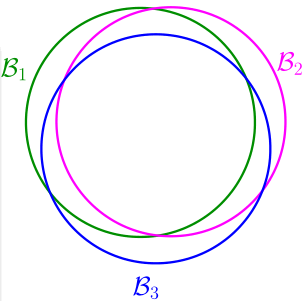• Why?

• $E_{out}(h_1) \approx E_{out}(h_2)$
• for most $\cal D$, $E_{in}(h_1) = E_{in}(h_2)$
• union bound over-estimating

• to account for overlap, can we group similar hypotheses by kind?

• How many lines are there?

• Effective number of lines

$N$ effective $(N)$
$1$ $2$
$2$ $4$
$3$ $8$
$4$ $14\ (<2^N)$
• maximum kinds of lines with respect to $N$ inputs $\mathbf{x}_1, \cdots, \mathbf{x}_N \Leftrightarrow$ effective numbers of lines

• must be $\le 2^N$
• finite grouping of infinitely-many lines $\in \cal H$
• wish:
• if

• $\text{effective}(N)$ can replace $M$ and
• $\text{effective}(N) \ll 2^N$
• learning possible with infinite lines.

QUIZ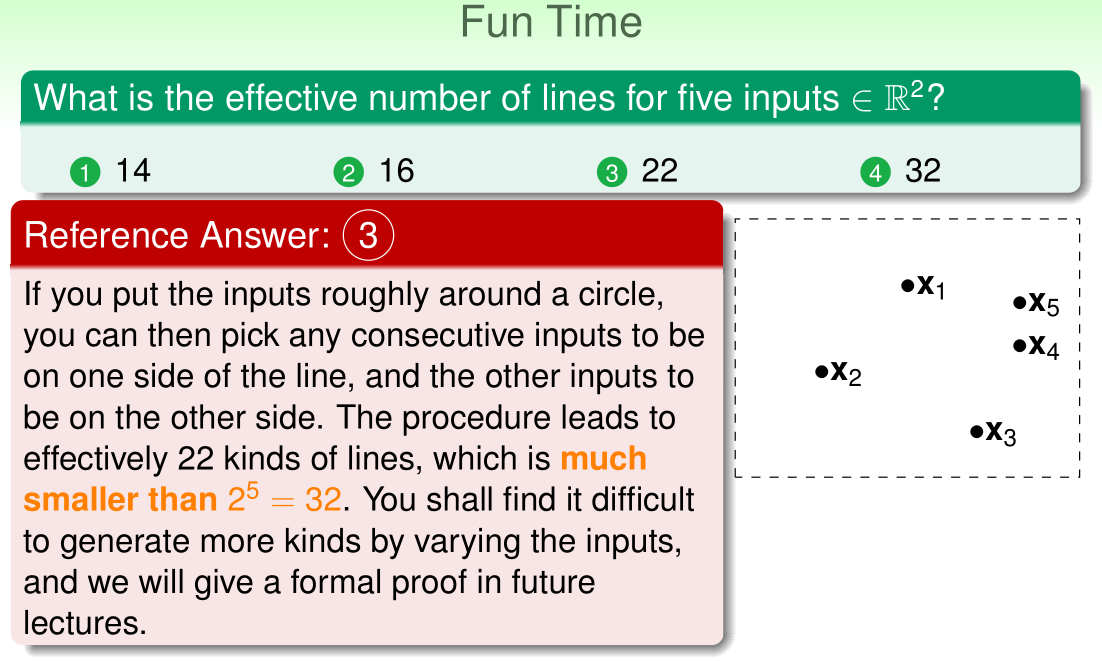#### 5.3 Effective Number of Hypothesis

• Dichotomies: mini-hypothesis

• call $h(\mathbf{x}_1, \dots, \mathbf{x}_N) = \left( h(\mathbf{x}_1), \dots, h(\mathbf{x}_N) \right) \in \lbrace {\color{Red}{\times}},{\color{Blue}{\circ}}\rbrace^N$

• a dichotomy: hypothesis limited to the eyes of $\mathbf{x}_1, \dots, \mathbf{x}_N$

• $\mathcal{H}(\mathbf{x}_1, \dots, \mathbf{x}_N)$: all dichotomies implemented by $\cal H$ on $\mathbf{x}_1, \dots, \mathbf{x}_N$

hypothesis $\cal H$ dichotomies $\mathcal{H}(\mathbf{x}_1, \dots, \mathbf{x}_N)$
e.g. all lines in $\Bbb{R}^2$ $\lbrace {\color{Blue}{\circ}}{\color{Blue}{\circ}}{\color{Blue}{\circ}}{\color{Blue}{\circ}},{\color{Blue}{\circ}}{\color{Blue}{\circ}}{\color{Blue}{\circ}}{\color{Red}{\times}},{\color{Blue}{\circ}}{\color{Blue}{\circ}}{\color{Red}{\times}}{\color{Red}{\times}},\dots\rbrace$
size possibly infinite upper bounded by $2^N$
• $\vert \mathcal{H}(\mathbf{x}_1, \dots, \mathbf{x}_N) \vert$: candidate for replacing $M$

• Growth function

• $\vert \mathcal{H}(\mathbf{x}_1, \dots, \mathbf{x}_N) \vert$: depends on inputs $(\mathbf{x}_1, \dots, \mathbf{x}_N)$

• growth function: remove dependence by taking $\max$ of all possible $(\mathbf{x}_1, \dots, \mathbf{x}_N)$

• finite, upper-bounded by $2^N$

• Growth function for positive rays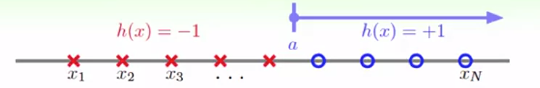• $\mathcal{X} = \Bbb{R}$ (one dimensional)
• $\cal H$ contains $h$, where each $h(x) = \text{sign}(x-a)$ for threshold $a$
• positive half of 1-D perceptrons
• one dichotomy for $a \in$ each spot $(x_n, x_{n+1})$: $m_{\cal H}(N) = N + 1$
• $(N+1) \ll 2^N$ when $N$ large!
• Growth function for positive intervals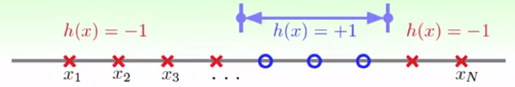• $\mathcal{X} = \Bbb{R}$ (one dimensional)

• $\cal H$ contains $h$, where each $h(x) = +1 \text{ iff } x \in [\mathcal{l}, r), -1$ otherwise

• one dichotomy for each interval kind:

• $\frac{1}{2}N^2+\frac{1}{2}N+1 \ll 2^N$ when $N$ large!

• Growth function for convex sets

• $\mathcal{X} = \Bbb{R}^2$ (two dimensional)

• $\cal H$ contains $h$, where each $h(x) = +1 \text{ iff$x$in a convex region}, -1$ otherwise

• one possible set of $N$ inputs: $\mathbf{x}_1, \dots, \mathbf{x}_N$ on a big circle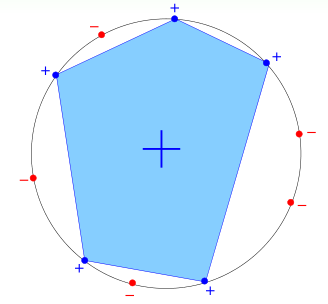• every dichotomy can be implemented by $\cal H$ using a convex region slightly extended from contour of positive inputs: $m_{\cal H} = 2^N$

• call this $N$ inputs shattered by $\cal H$

• $m_{\cal H}(N) = 2^N \Leftrightarrow$ exists $N$ inputs that can be shattered

QUIZ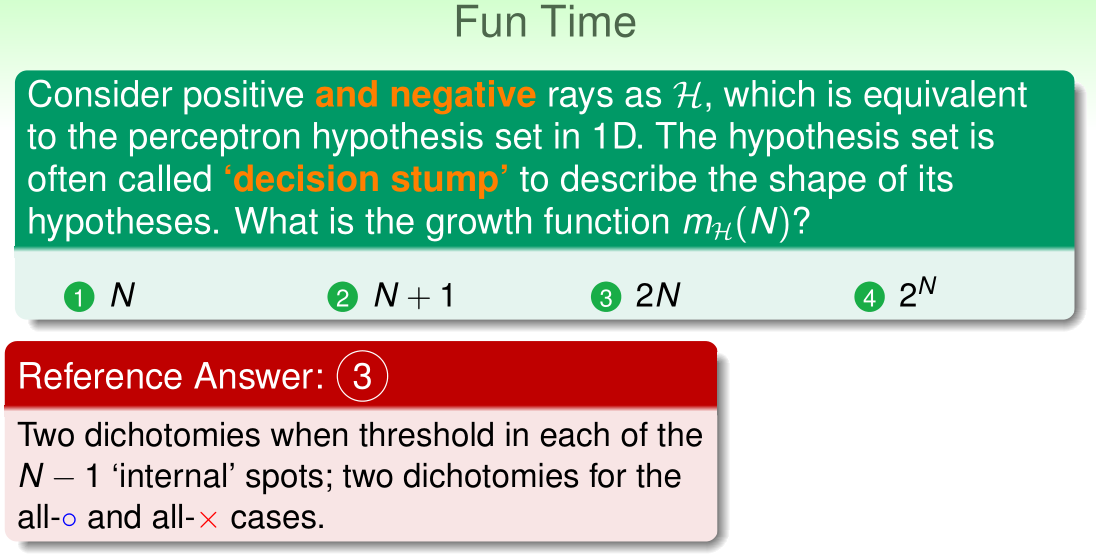#### 5.4 Break Point

• The four growth functions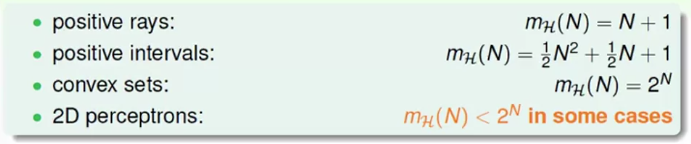• what if $m_{\cal H}(N)$ replaces $M$?

• polynomial: good :+1:
• for 2D or general perceptron, $m_{\cal H}(N)$ polynomial (good)?

• Break point of $\cal H$

• what do we know about 2D perceptrons now?
• three inputs: exists shatter
• four inputs: for all no shatter
• if no $k$ inputs can be shattered by $\cal H$, call $k$ a break point for $\cal H$
• $m_{\cal H} < 2^k$
• $k+1, k+2, \dots$ also break points!
• will study minimum break point $k$
• The four beak points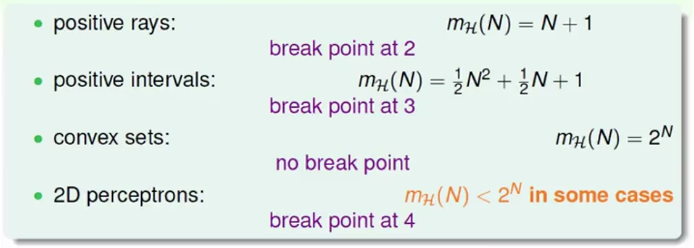• conjecture:
• no break point: $m_{\cal H} = 2^N$ (sure!)
• break point $k$: $m_{\cal H} = O(N^{k-1})$
• exited? wait for next lecture ;-)
QUIZ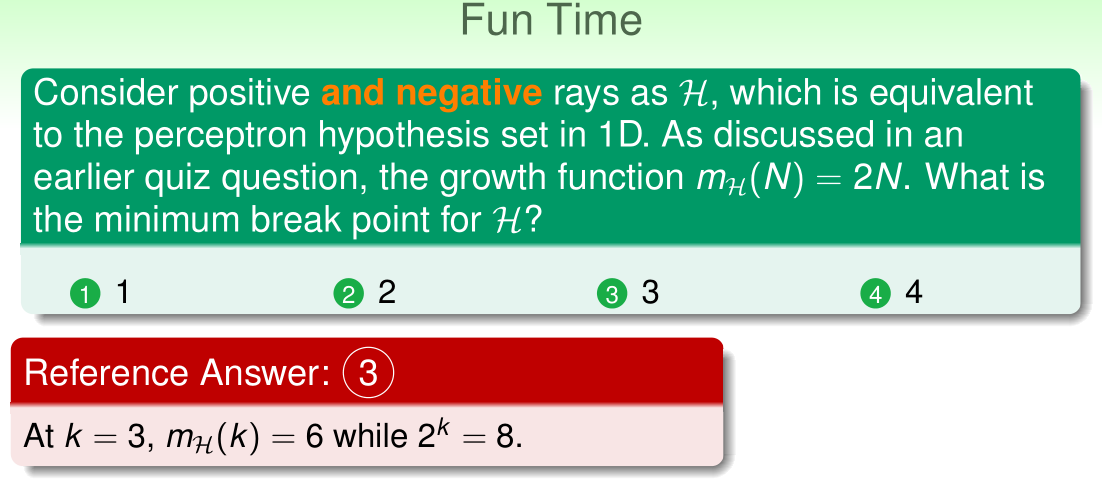## 6 Theory of Generalization

Test error can approximate training error if there is enough data and growth function does not grow too fast …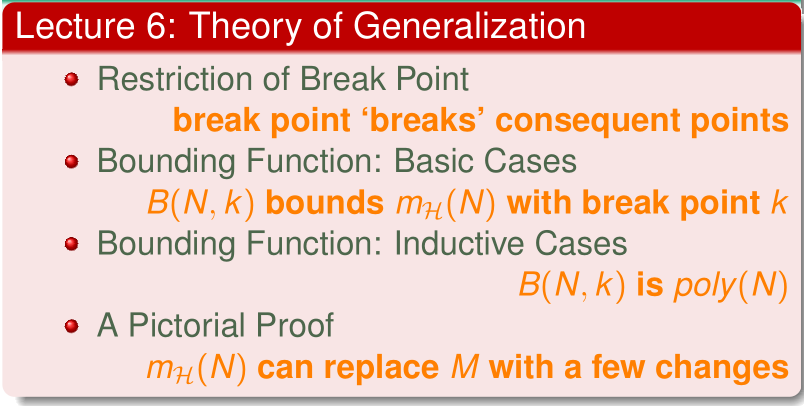#### 6.1 Restiction of Break Point

• The four break points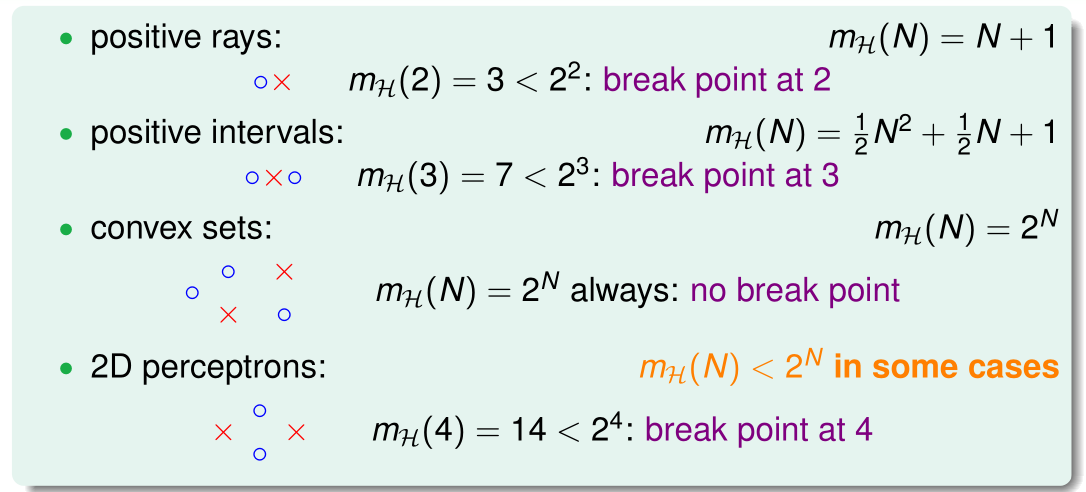• growth function $m_{\cal H}(N)$: max number of dichotomies
• break point $k \Rightarrow$ break point $k + 1, \dots$
• what else?
• Restriction of break point

• what must be true when minimum break point $k = 2$
• $N = 1$: every $m_{\cal H}(N) = 2$ by definition
• $N = 2$: every $m_{\cal H}(N) < 4$ by definition
• (so maximum possible = 3)
• $N = 3$: maximum possible $= 4 \ll 2^3$
• break point $k$ restricts maximum possible $m_{\cal H}(N)$ a lot for $N > k$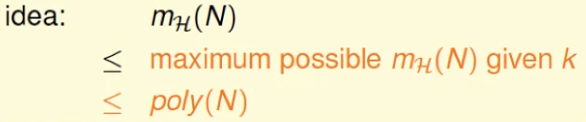QUIZ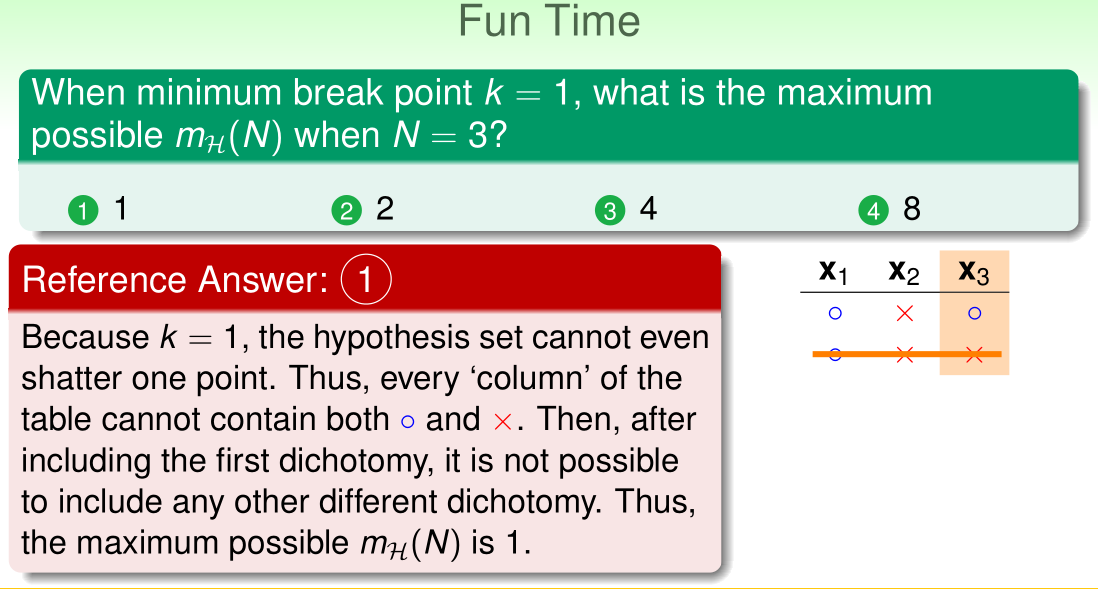#### 6.2 Bounding Function: Basic Cases

• Bounding function $B(N, k)$: maximum possible $m_{\cal H}(N)$ when break point $= k$
• combinatorial quantity:
• maximum number of length-$N$ vectors with $({\color{Red}{\times}},{\color{Blue}{\circ}})$ while “no shatter” any length-$k$ subvectors
• irrelevant of the details of $\cal H$
• e.g. $B(N, 3)$ bounds both
• positive intervals ($k = 3$)
• 1D perceptrons ($k = 3$)
• new goal: $B(N, k) \le \text{poly}(N)$ ?
QUIZ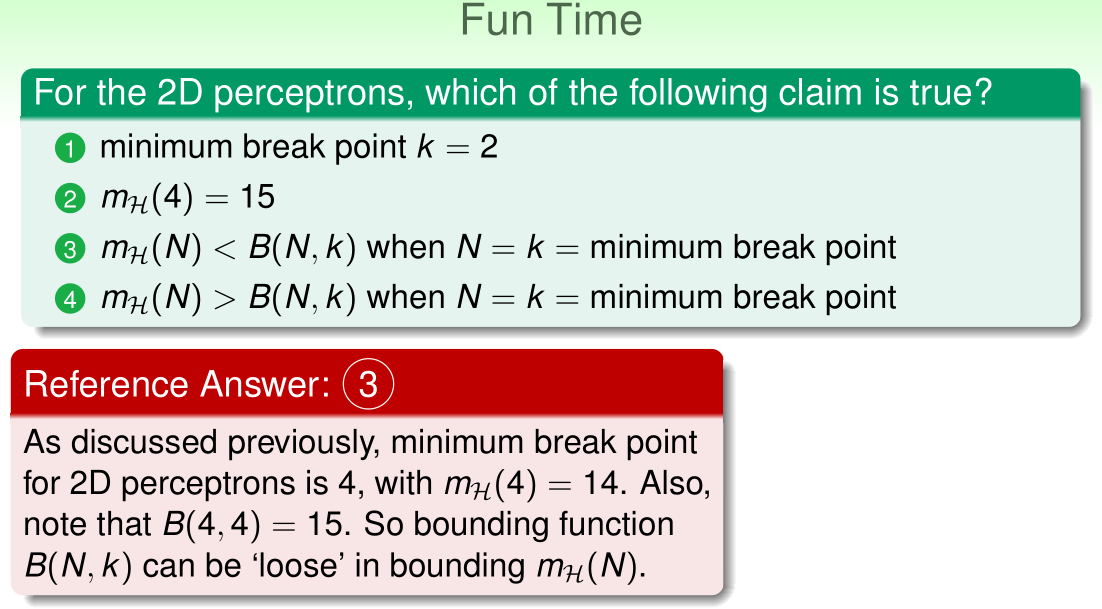#### 6.3 Bounding Function: Inductive Cases

• Table of bounding funciton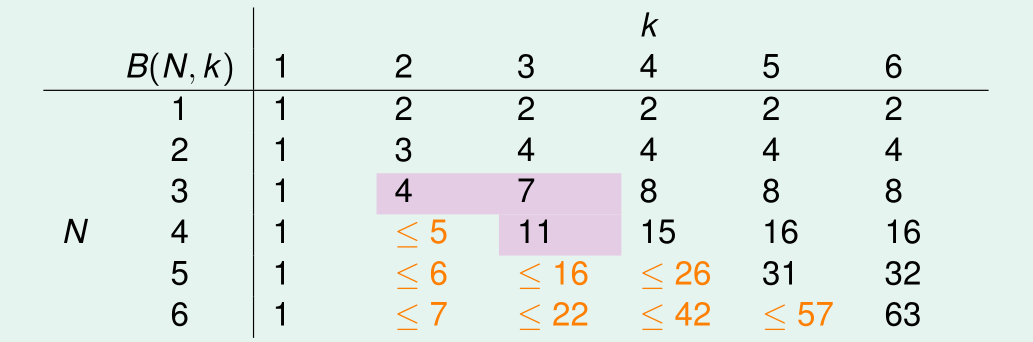• Known
• $B(N, 1) = 1$ (see previous quiz)
• $B(N, k) = 2^N \text{ for } N < k$
• $B(N, k) = 2^N-1 \text{ for } N = k$
• $B(N, k) \le B(N-1, k) +B(N-1, k-1)$
• (actually “$=$”)
• Now have upper bound of bounding function
• Bounding function: the theorem

• simple induction using boundary and inductive formula
• for fixed $k$, $B(N, k)$ upper bounded by $\text{poly}(N)$
• $\Rightarrow m_{\cal H}(N)$ is $\text{poly}(N)$ if break points exists
• ”$\le$” can be “$=$” actually
• The three break points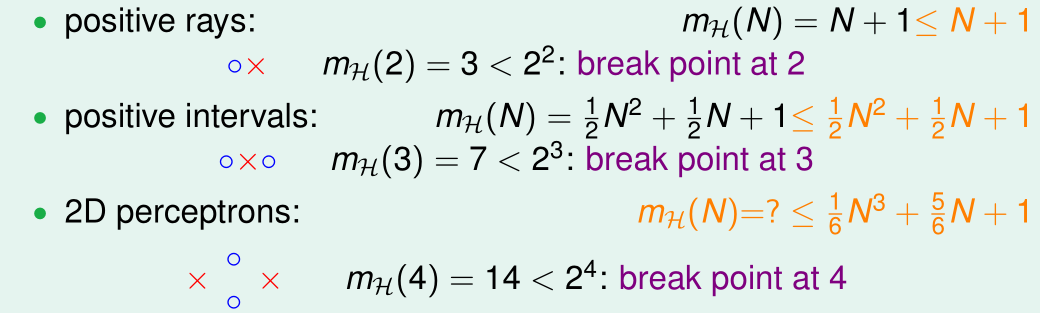QUIZ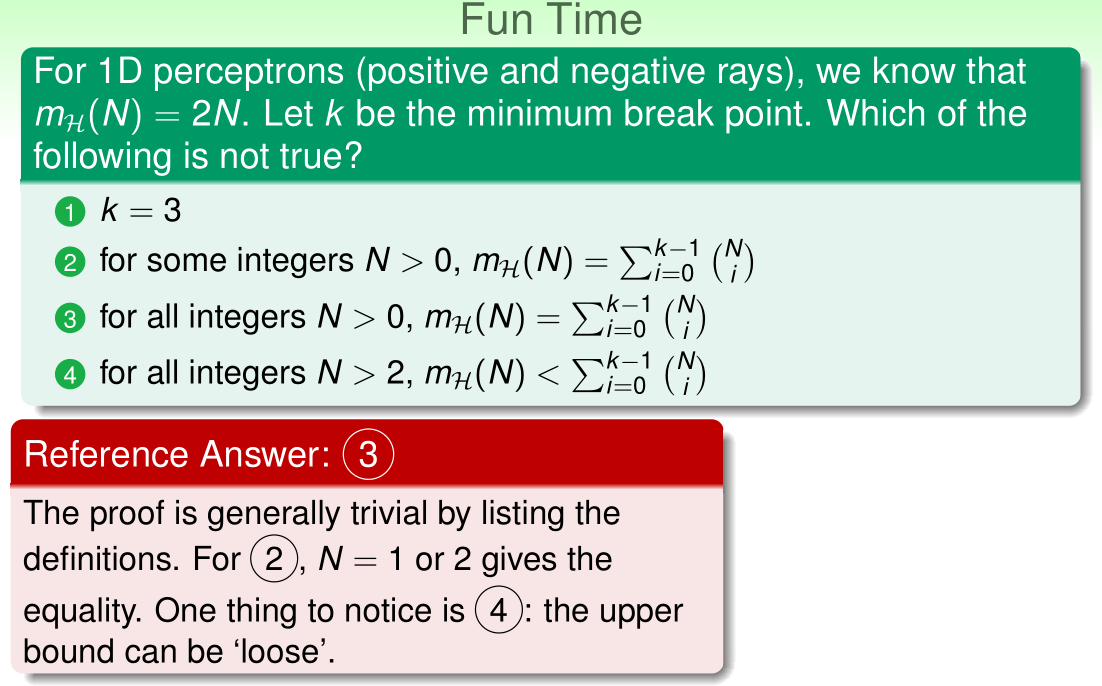#### 6.4 A Pictorial Proof

• BAD Bound for General $\cal H$

• want:

• actually, when $N$ is large enough:

• next: sketch of proof

• Step 1: Replace $E_{out}$ by $E_{in}^{\prime}$

• $E_{\text {in}}(h)$ finitely many, $E_{\text {out}}(h)$ infinitely many

• replace the evil $E_{\text {out}}(h)$ first
• how? sample verification set $\cal D^{\prime}$ of size $N$ to calculate $E_{\text {in}}^{\prime}$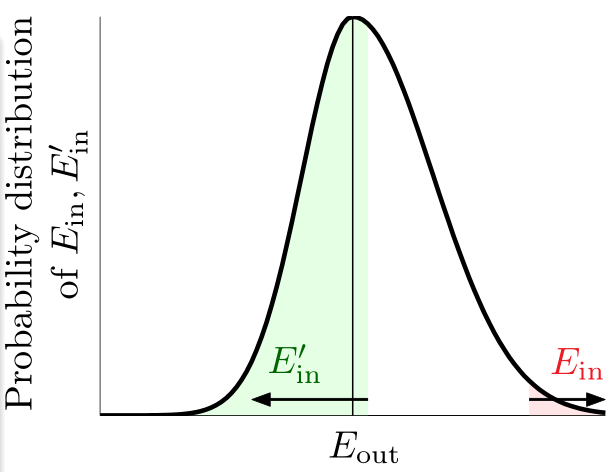• BAD $h$ of $E_{\text {in}} - E_{\text {out}}$

• $\stackrel{\text { probably }}{\Longrightarrow}$ BAD $h$ of $E_{\text {in}} - E_{\text {in}}^{\prime}$
• Step 2: Decompose $\cal H$ by Kind

• $E_{\text {in}}$ with $\cal D$, $E_{\text {in}}^{\prime}$ with $\cal D^{\prime}$
• now $m_{\cal H}$ comes to play
• how? infinite $\cal H$ becomes $\vert \mathcal{H}(\mathbf{x}_1, \dots, \mathbf{x}_N)\vert$ kinds
• union bound on $m_{\cal H}(2N)$ kinds
• Step 3: Use Hoeffding without Replacement

• consider bin of $2N$ examples, choose $N$ for $E_{\text{in}}$,leave others for $E_{\text{in}}^{\prime}$
• $\vert E_{\text{in}} - E_{\text{in}}^{\prime} \vert > \frac{\epsilon}{2} \Leftrightarrow \left \vert E_{\text{in}} - \frac{E_{\text{in}}+E_{\text{in}}^{\prime}}{2} \right\vert > \frac{\epsilon}{4}$
• so? just similar bin, smaller $\epsilon$, and Hoeffding without replacement
• That’s All! $\Rightarrow$ Vapnik-Chervonenkis( VC) bound:

• replace $E_{\text{out}}$ by $E_{\text{in}}^{\prime}$
• decompose $\cal H$ by kind
• use Hoeffding without replacement
• 2D perceptrons:
• break point? 4
• $m_{\cal H}(N)$? $O(N^3)$
• learning with 2D perceptrons feasible! 🎉
QUIZ## 7 The VC Dimension

Learning happens if there is finite model complexity (called VC dimension), enough data, and low training error …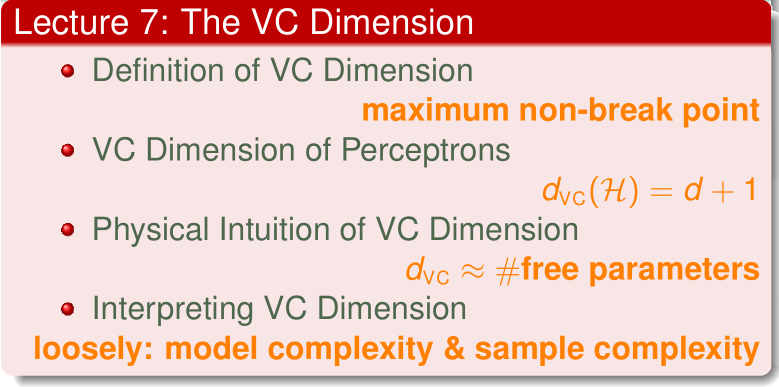#### 7.1 Definition of VC Dimension

• Recap: More on Growth Function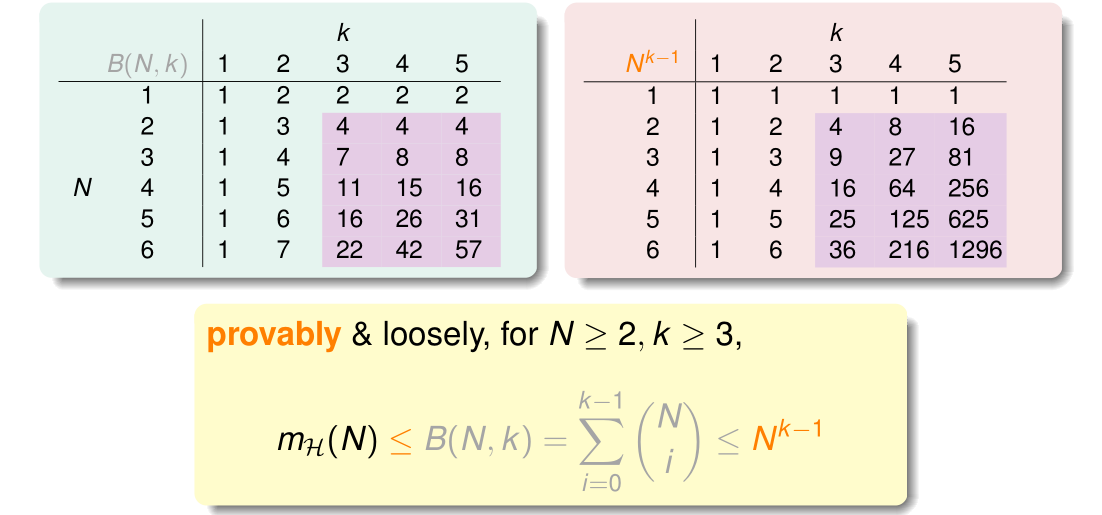• Recap: More on Vapnik-Chervonenkis(VC) Bound• if:
• ① $m_{\cal H}(N)$ breaks at $k$ (good $\cal H$)
• ② $N$ large enough (good $\cal D$)
• $\Rightarrow$ probably generalized $E_{out} \approx E_{in}$,
• and if:
• ③ $\cal A$ picks a $g$ with small $E_{in}$ (good $\cal A$)
• $\Rightarrow$ probably learned! 🎉
• VC Dimension

• the formal name of maximum non-break point

• Definition: VC dimension of $\cal H$, denoted $d_{\text{VC}}(\cal H)$ is

• the most inputs $\cal H$ that can shatter
• $d_{\text{VC}} = \min(k) - 1$
• $N \leq d_{\text{VC}} \Rightarrow$

• $\cal H$ can shatter some $N$ inputs
• $N > d_{\text{VC}} \Rightarrow$

• $N$ is a break point for $\cal H$
• $\text{if } N \ge 2, d_{\text{VC}} \ge 2, m_{\cal H} \leq N^{d_{\text{VC}}}$

• The Four VC Dimensions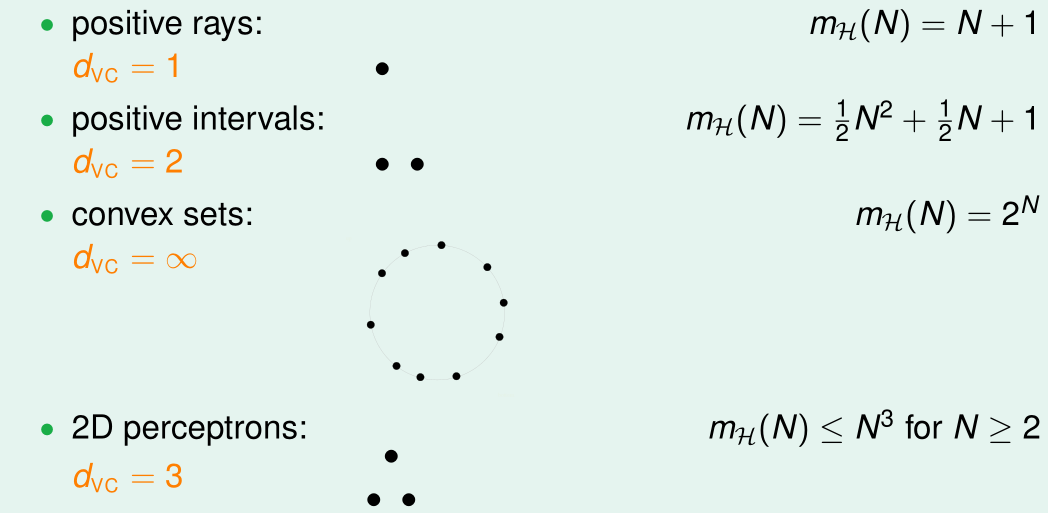• VC Dimension and Learning

• good $\Rightarrow$ finite $d_{\text{VC}}$ $\Rightarrow$ $g$ “will” generalize $(E_{out}(g) \approx E_{in}(g))$
• regardless of learning algorithm $\cal A$
• regardless of input distribution $P$
• regardless of target function $f$
QUIZ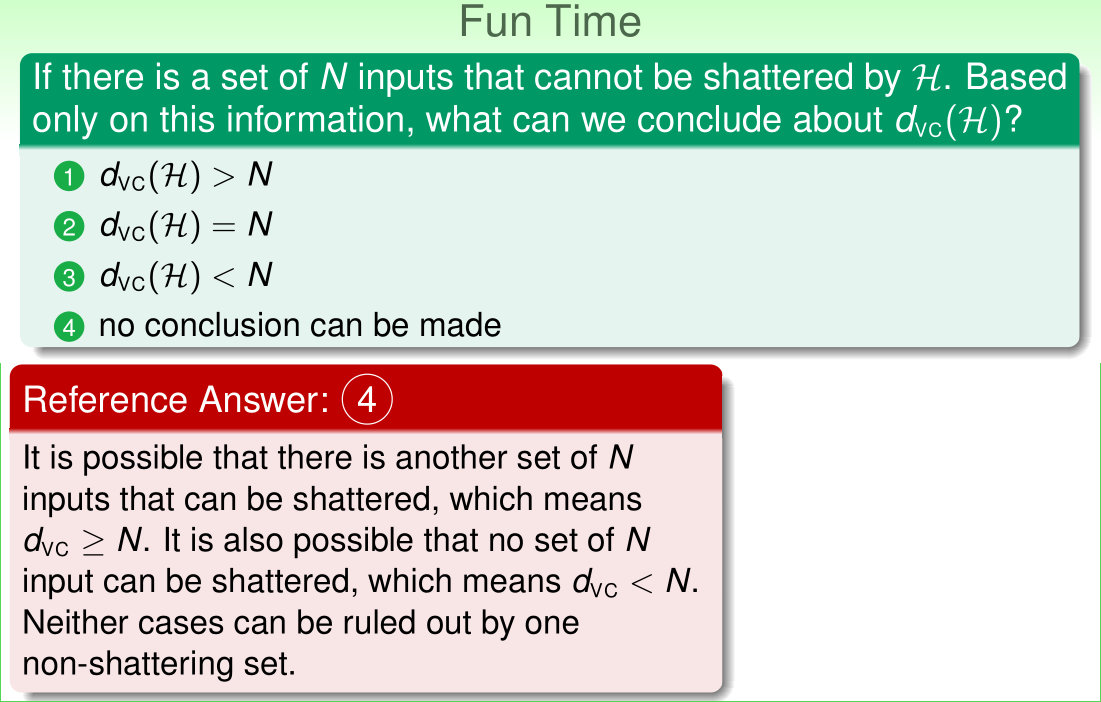#### 7.2 VC Dimension of Perceptrons

• 2D PLA Revisited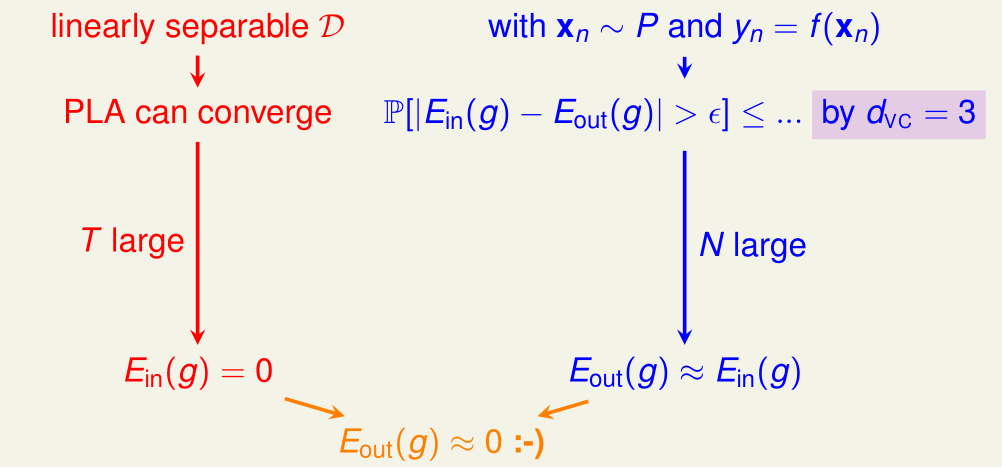• general PLA for $\mathbf{x}$ with more that 2 features?
• VC Dimension of Perceptrons

• 1D perceptron (pos/neg rays): $d_{\text{VC}} = 2$
• 2D perceptron: $d_{\text{VC}} = 3$
• $d$-D perceptrons: $d_{\text{VC}} \mathop{=}^{?}d+1$
EXTRA QUIZ• $d_{\text{VC}} \ge d + 1$

• There are some $d+1$ inputs we can shatter

• some “trivial” inputs:

• note: $\mathbf{X}$ invertible

• to shatter …

• for any $\bf y \in \Bbb{R}^{d+1}$, find $\mathbf{w}$ such that
• $\text{sign}(\mathbf{Xw})=\mathbf{y} \Leftarrow \bf{Xw=y} \stackrel{X\text{ invertible!}}{\Longleftrightarrow} w= X^{-1}y$
EXTRA QUIZ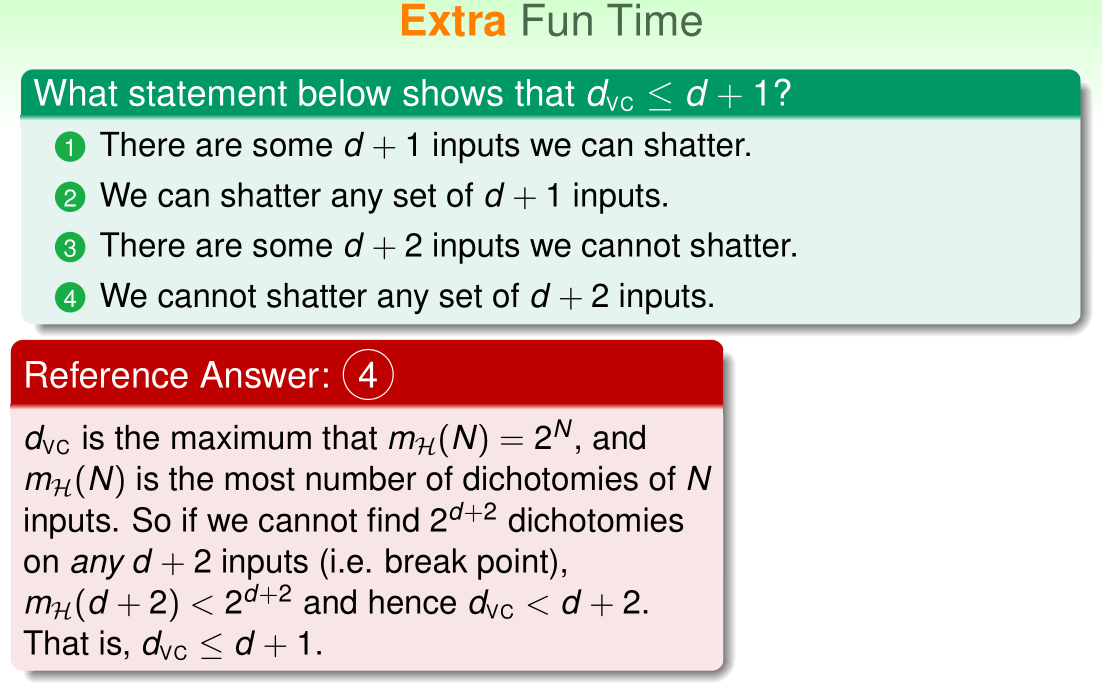• $d_{\text{VC}} \ge d+1$

• A 2D special case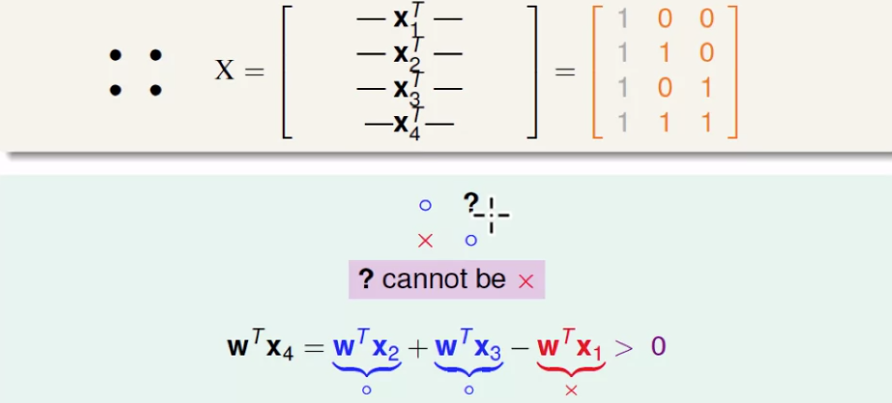• $d$-D general case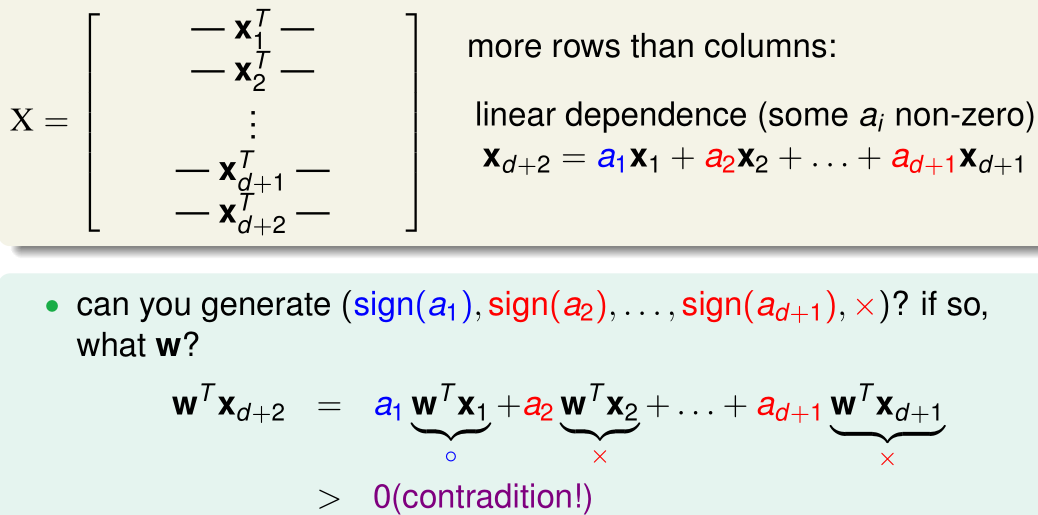• general $\bf X$ no-shatter $\Rightarrow d_{\text{VC}}\le d+1$

QUIZ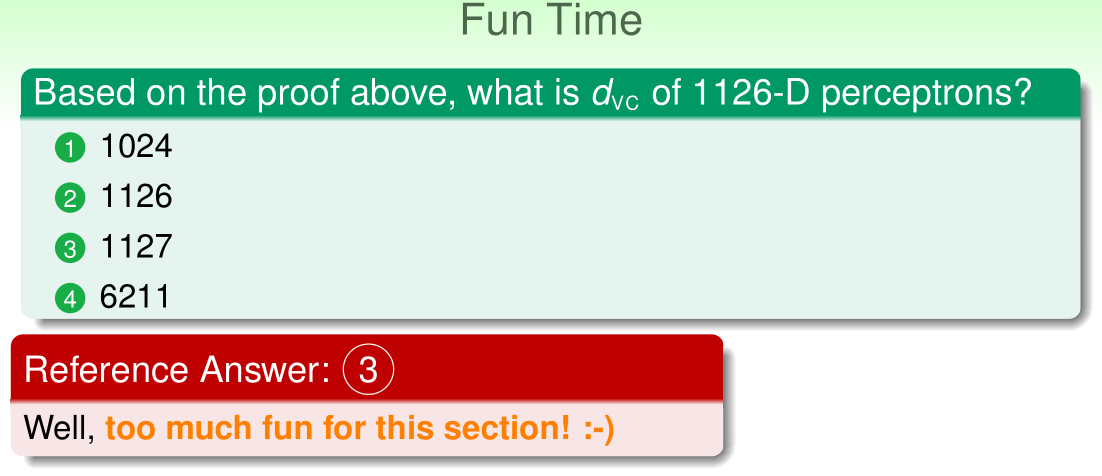#### 7.3 Physical Intuition of VC Dimension

• Degrees of Freedom

• hypothesis parameters $\mathbf{w}=(w_0, w_1, \dots, w_d)$: creates degrees of freedom
• hypothesis quantity $M = \vert \mathcal{H} \vert$: “analog” degrees of freedom
• hypothesis power $d_{\text{VC}}=d+1$: effective “binary” degrees of freedom
• $d_{\text{VC}}(\cal H)$: powerfulness of $\cal H$
• Two Old Friends

• Positive rays ($d_{\text{VC}}=1$)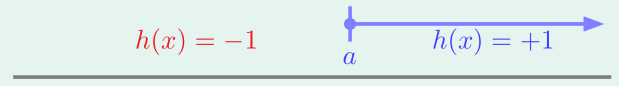• free parameters: $a$
• Positive intervals ($d_{\text{VC}}=2$):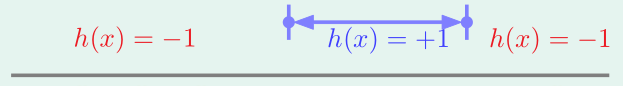• free parameters: $\mathcal{l}, r$
• pratical rule of thumb: $d_{\text{VC}} \approx \text{#free parameters}$ (but not always)

• $M$ and $d_{\text{VC}}$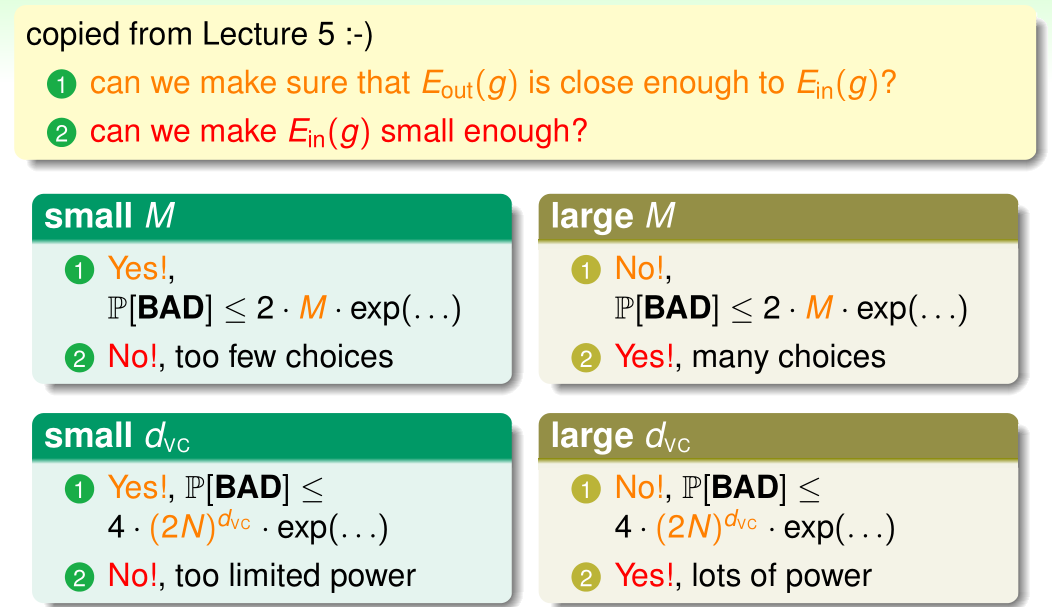• using the right $d_{\text{VC}}$ (or $\cal H$) is important
QUIZ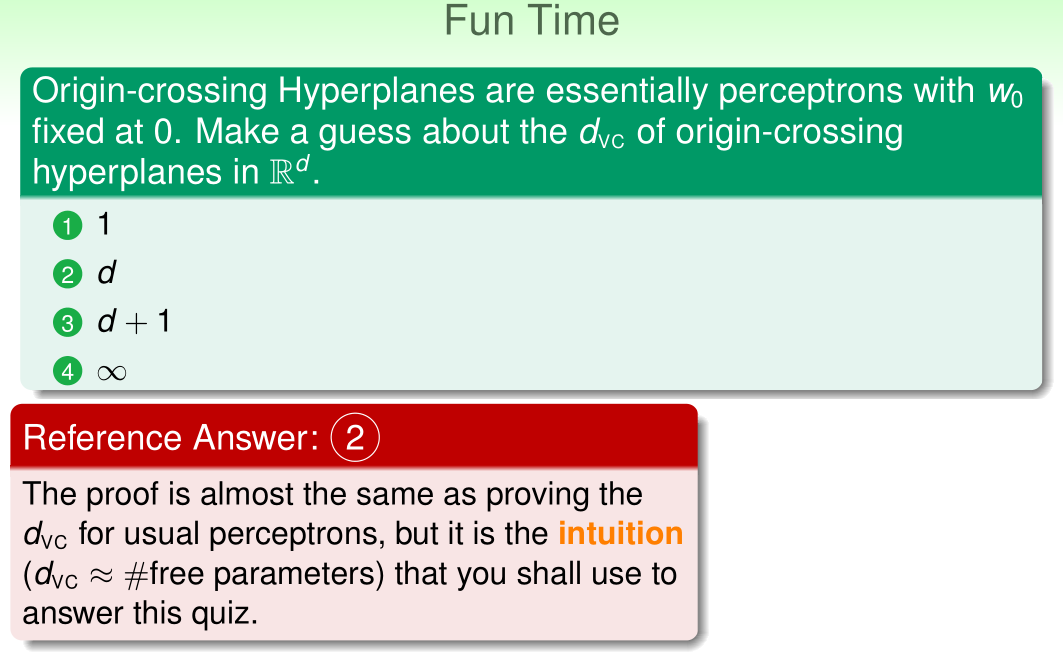#### 7.4 Interpreting VC Dimension

• VC Bound Rephrase: Penalty for Model Complexity

• For any ${\color{Red}{g}={\color{Red}{\cal A}}}({\color{Blue}{\cal D}}) \in \mathcal {\color{Orange}{H}}$ and statistical large $\cal D$, for $d_{\text{VC}} \ge 2$:

• Rephrase: with prbability $\ge 1 - \delta$, GOOD: $\left\vert E_{\text {in }}(g)-E_{\text {out }}(g)\right\vert \leq \epsilon$

• generalization error $\left\vert E_{\text {in }}(g)-E_{\text {out }}(g)\right\vert \le \sqrt{\frac{8}{\color{Blue} N } \ln \left(\frac{4{\color{Orange} (2 N)^{d_{\mathrm{VC}}}} }{\color{Purple} \delta }\right)}$

• $E_{\text {in }}(g)- \sqrt{\frac{8}{\color{Blue} N} \ln \left(\frac{4{\color{Orange} (2 N)^{d_{\mathrm{VC}}}} }{\color{Purple} \delta} \right)} \le E_{\text {out }}(g) \le E_{\text{in}}(g) + \sqrt{\frac{8}{\color{Blue} N} \ln \left(\frac{4{\color{Orange} (2 N)^{d_{\mathrm{VC}}}} }{\color{Purple} \delta} \right)}$

• $\underbrace{\sqrt{\cdots}}_{\Omega(N, \mathcal{H}, \delta)}$： penalty for model complexity

• THE VC Message

• with a high probability, $E_{\text {out }}(g) \le E_{\text{in}}(g) + \underbrace{\sqrt{\frac{8}{\color{Blue} N} \ln \left(\frac{4{\color{Orange} (2 N)^{d_{\mathrm{VC}}}} }{\color{Purple} \delta }\right)}}_{\Omega(N, \mathcal{H}, \delta)}$
•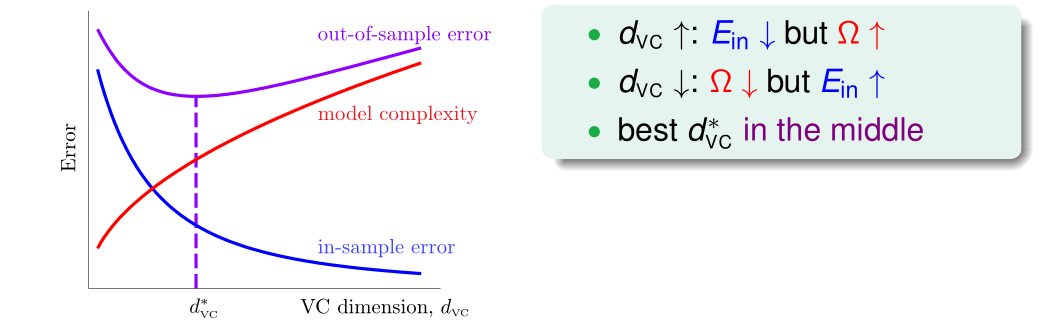• powerful $\cal H$ not always good!
• VC Bound Rephrase: Sample Complexity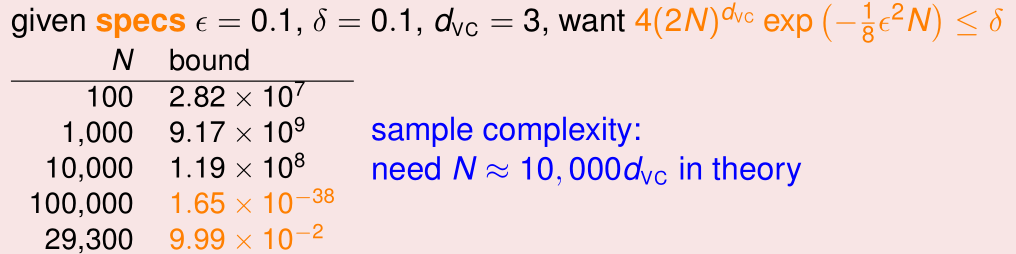• practical rule of thumb:
• $N \approx 10 d_{\text{VC}}$ often enough!
• Looseness of VC Bound

• theory: $N \approx 10,000\ d_{\text{VC}}$; practice: $N \approx 10\ d_{\text{VC}}$

• Why?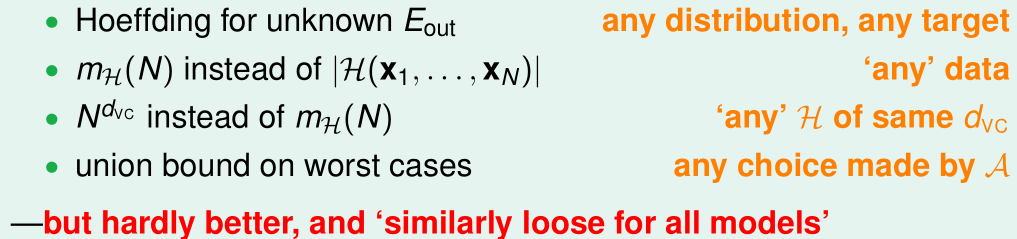• philosophical message of VC bound: important for improving ML

QUIZ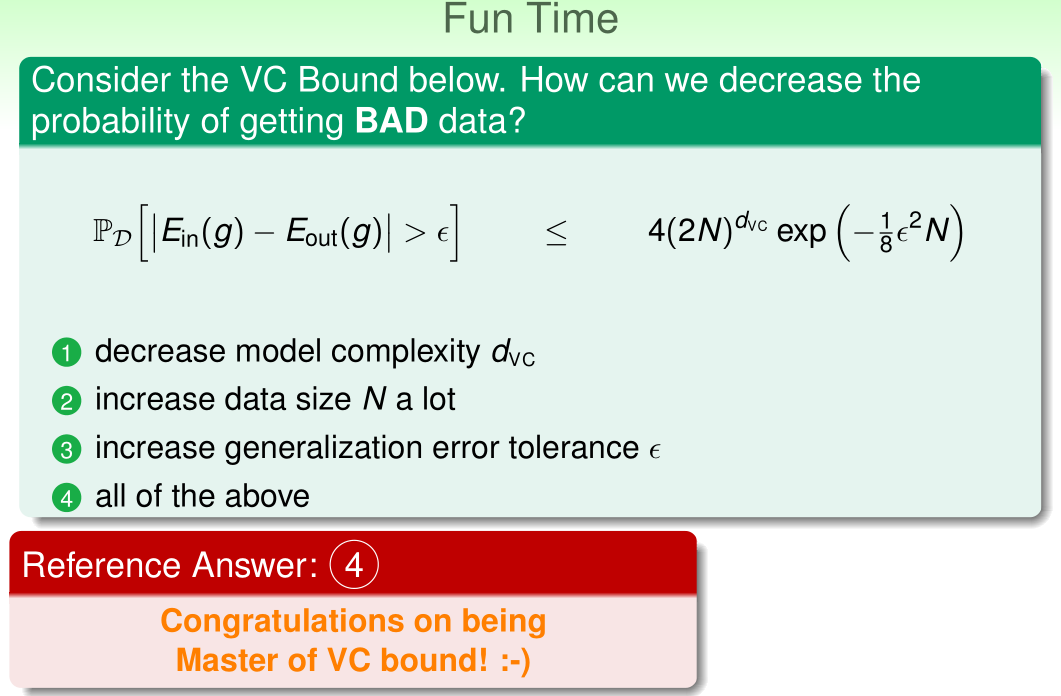## 8 Noise and Error

Learning can still happen within a noisy environment and different error measures …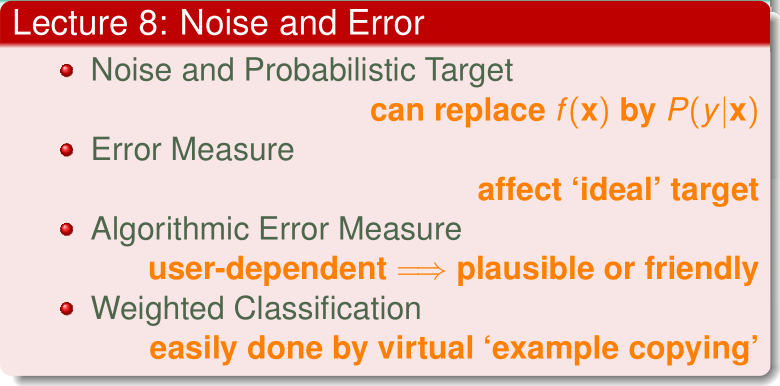#### 8.1 Noise and Probabilistic Target

• Recap: The Learning Flow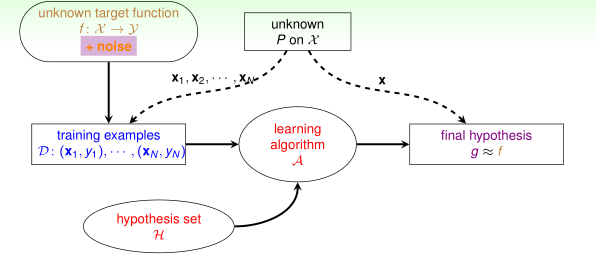• what if there is noise?
• Noise

• Probabilistic Marbles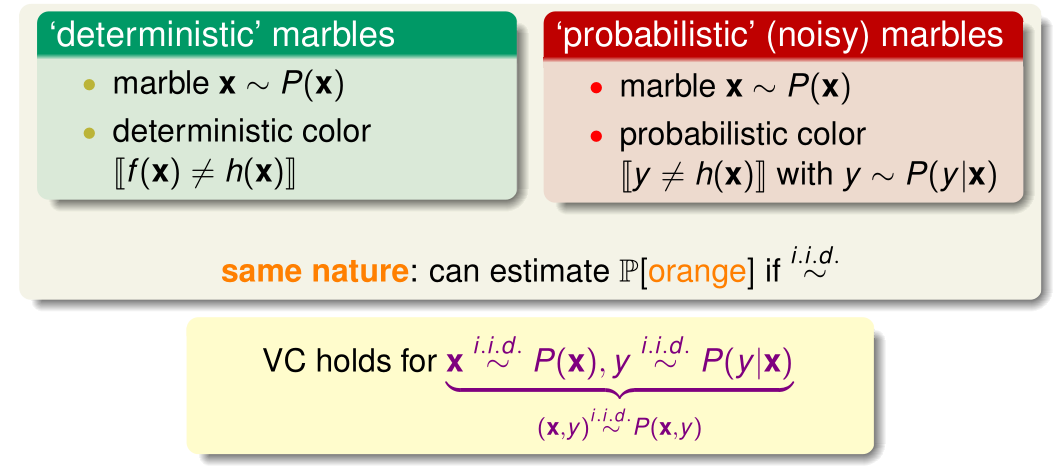• Target Distribution $P(y\vert\mathbf{x})$: characterizes behavior of “mini-target” on one $\bf x$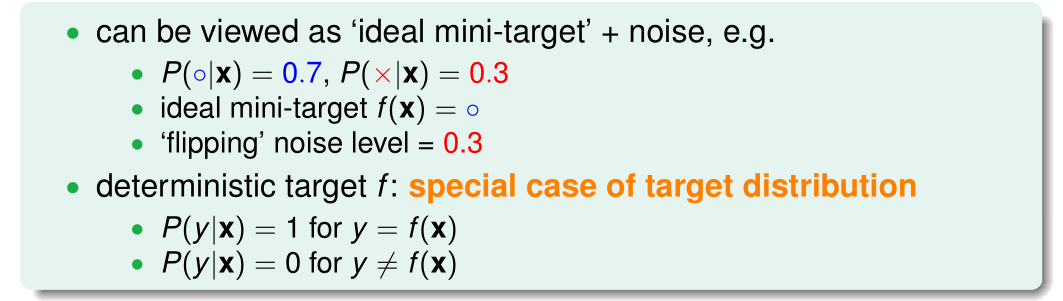• goal of learning:
• predict ideal mini-target (w.r.t. $P(y\vert \mathbf{x})$) on often-seen inputs (w.r.t $P(\mathbf{x})$)
• The New Learning Flow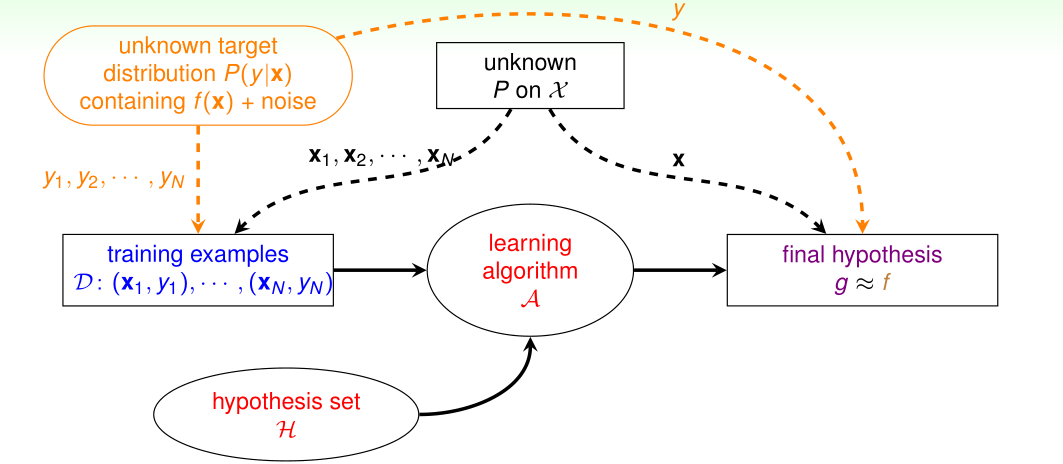QUIZ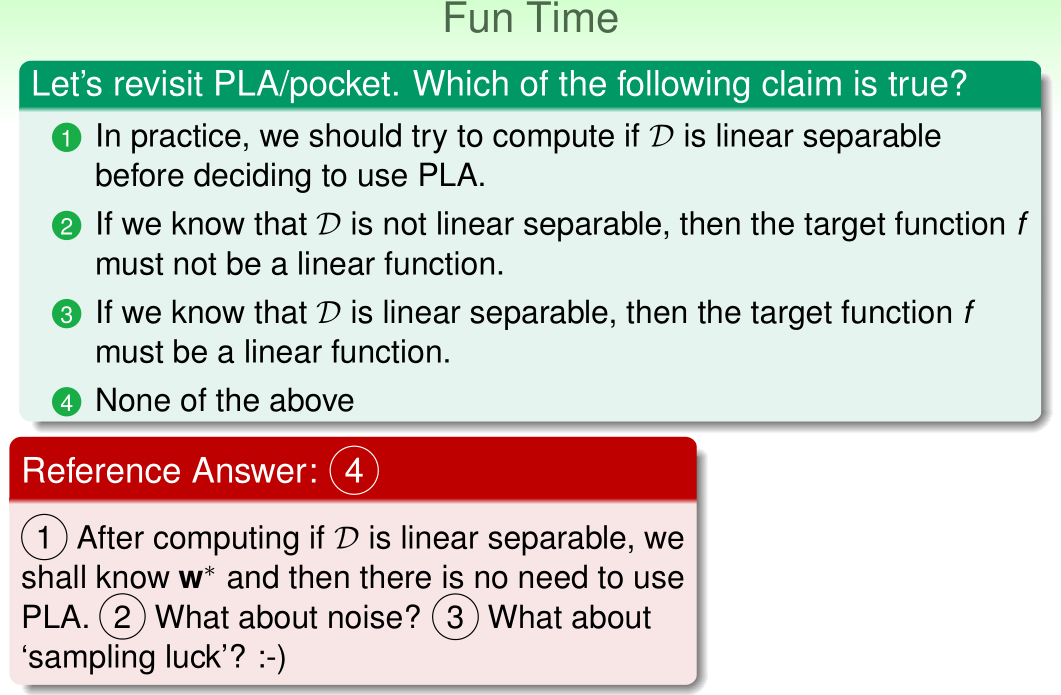#### 8.2 Error Measure

• Error Measure: final hypothesis $g \approx f$

• how well? previously, considered out-of-sample measure

• more generally, error measure $E(g, f)$
• naturally considered
• out-of-sample: averaged over unknown $\bf x$
• pointwise: evaluated on one $\bf x$
• classification: $[\text{prediction} \ne \text{target}]$
• classification error often also called “0/1 error”
• Pointwise Error Measure

• can often express $E(g,f)$ = averaged $\mathop{\text{err}}(g(\mathbf{x}),f(\mathbf{x}))$, like

• $\text{err}$: called pointwise error measure
• in-sample:

• out-of-sample:

• will mainly consider pointwise $\operatorname{err}$ for simplicity

• Two Important Pointwise Error Measure: $\operatorname{err}(g(\mathbf{x}), f(\mathbf{x})) = \operatorname{err}(\tilde{y}, y)$

• 0/1 error: $\operatorname{err}(\tilde{y}, y)=[\tilde{y} \neq y]$
• correct or incorrect?
• often for classification
• squared error: $\operatorname{err}(\tilde{y}, y)=(\tilde{y}-y)^{2}$
• how far is $\tilde{y}$ from $y$?
• often for regression
• how does $\operatorname{err}$ “guide” learning?
• Ideal Mini-Target

• interplay between noise and error

• $P(y\vert \mathbf{x})$ and $\text{err}$ define ideal mini-target $f(\mathbf{x})$
• e.g.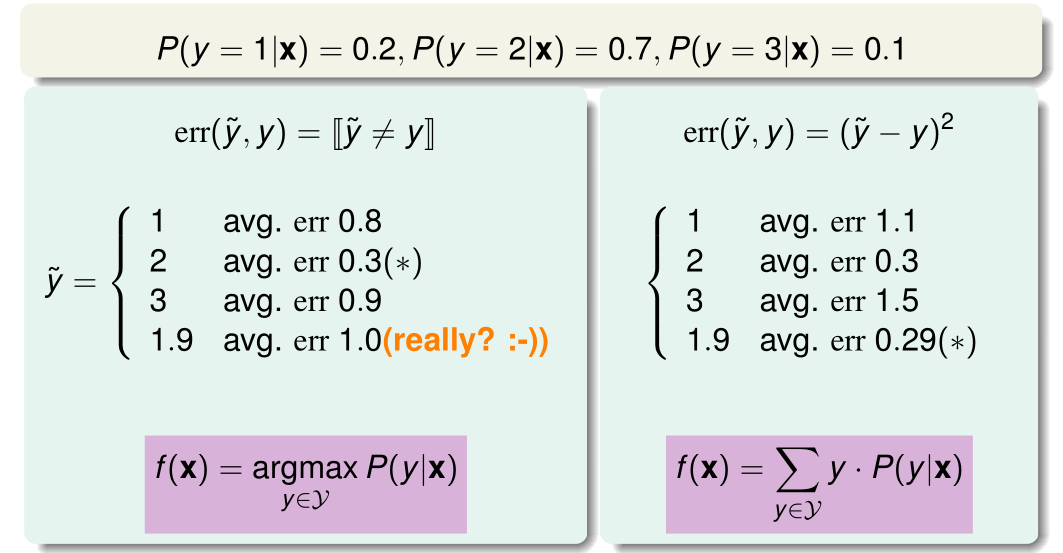• Learning Flow with Error Measure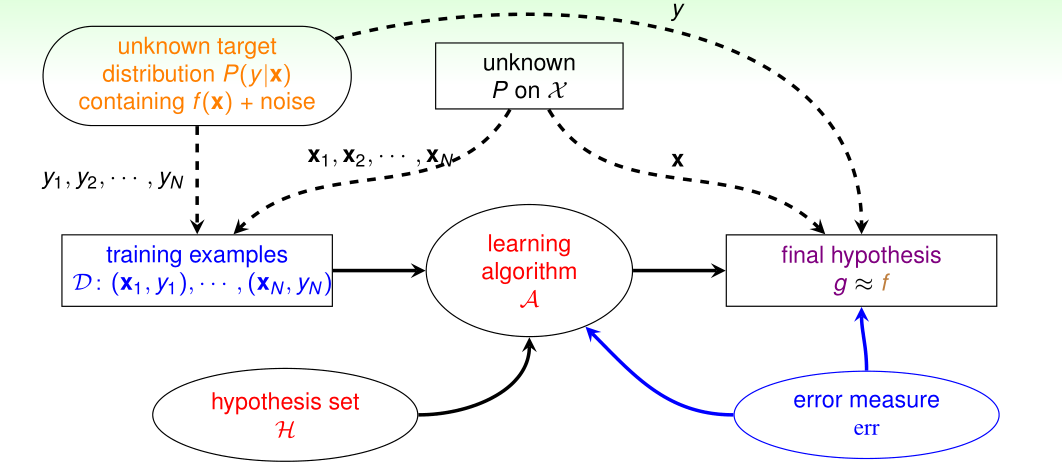• extended VC theory/”philosophy” works for most $\cal H$ and $\text{err}$
QUIZ#### 8.3 Algorithmic Error Measure

• Choice of Error Measure• two types of error: false accept and false reject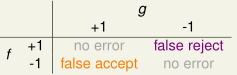• 0/1 error penalizes both types equally

• Fingerprint verification for supermarket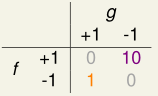• supermarket: fingerprint for discount
• false reject

: very unhappy customer, lose future business

• false accept

: give away a minor discount, intruder left fingerprint

• Fingerprint verification for CIA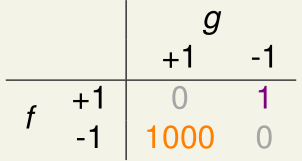• CIA: fingerprint for entrance
• false accept

: very serious consequences!

• false reject

: unhappy employee, but so what?

• Take-home Message for Now: $\text{err}$ is application/user-dependent

• Algorithmic Error Measures $\widehat{\text{err}}$
• true: just $\text{err}$
• plausible:
• 0/1: minimum “flipping noise”—NP-hard to optimize, remember?
• squared: minimum Gaussian noise
• friendly: easy to optimize for $\cal A$
• closed-form solution
• convex objective function
• $\widehat{\text{err}}$: more in next lectures
• Learning Flow with Algorithmic Error Measure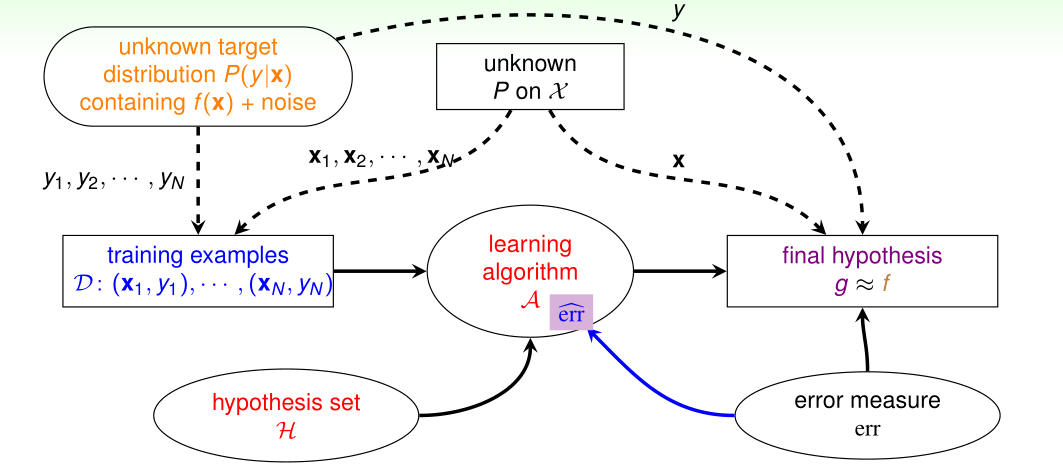QUIZ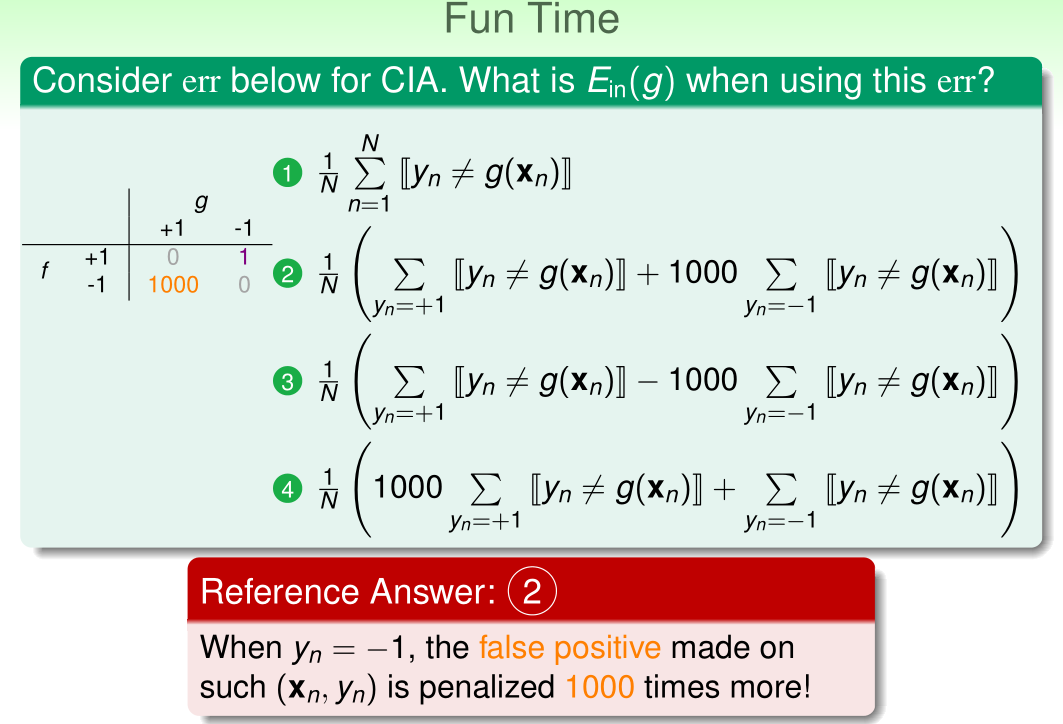#### 8.4 Weighted Classification

• Weighted Classification

• CIA cost (error, loss) matrix• out-of-sample

• in-sample

• weighted classification: different “weight” for different $(\mathbf{x},y)$

• Minimizing $E_{\text{in}}$ for Weighted Classification

• Naïve Thoughts
• PLA: doesn’t matter if linear separable
• pocket: modify pocket-replacement rule
• if $\mathbf{w}_ {t+1}$ reaches smaller $E_ {\mathrm{in}}^{\mathrm{w}}$ than $\hat{\mathbf{w}}$, replace $\hat{\bf w}$ by $\mathbf{w}_ {t+1}$
• But
• pocket: some guarantee on $E_{\mathrm{in}}^{0/1}$
• modified pocket: similar guarantee on $E_{\mathrm{in}}^{\mathrm{w}}$?
• Systematic Route: Connect $E_{\mathrm{in}}^{\mathrm{w}}$ and $E_{\mathrm{in}}^{\mathrm{0/1}}$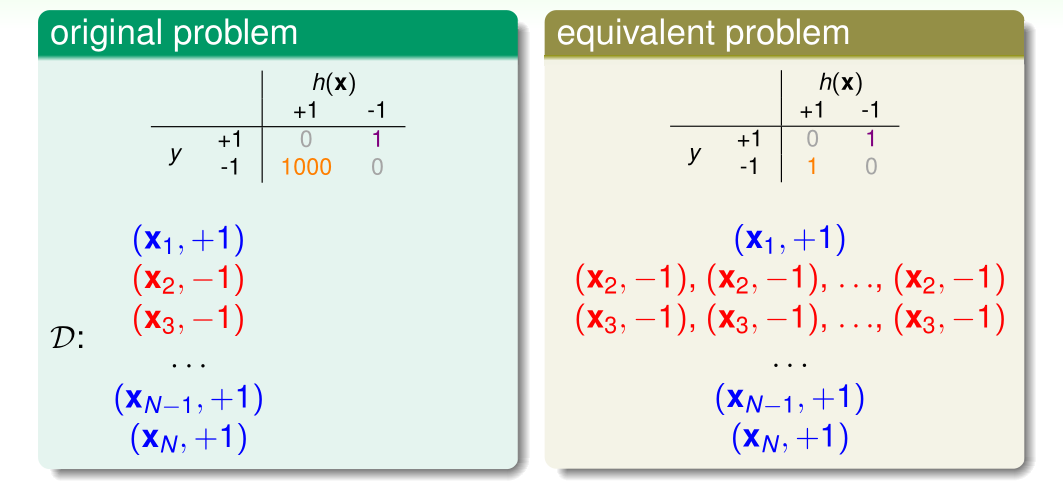• after copying “-1” examples 1,000 times, $E_{\mathrm{in}}^{\mathrm{w}} \text{ for LHA} \equiv E_{\mathrm{in}}^{\mathrm{0/1}} \text{ for RHS}$!
• Weighted Pocket Algorithm

• using “virtual copying”, weighted pocket algorithm include:
• weighted PLA:
• randomly check “-1” example mistakes with 1000 times more probability
• weighted pocket replacement:
• if $\mathbf{w}_ {t+1}$ reaches smaller $E_ {\mathrm{in}}^{\mathrm{w}}$ than $\hat{\mathbf{w}}$, replace $\hat{\bf w}$ by $\mathbf{w}_ {t+1}$
• systematic route (called “reduction”):
• can be applied to many other algorithms!
QUIZ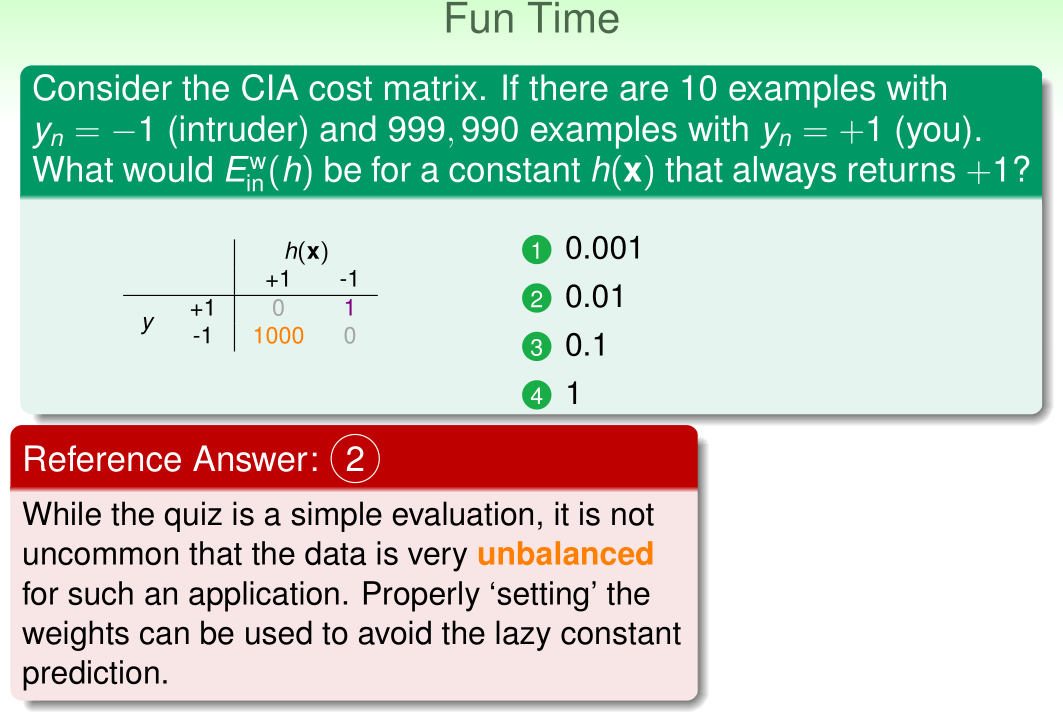Thistledown